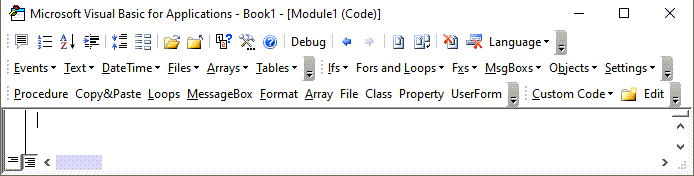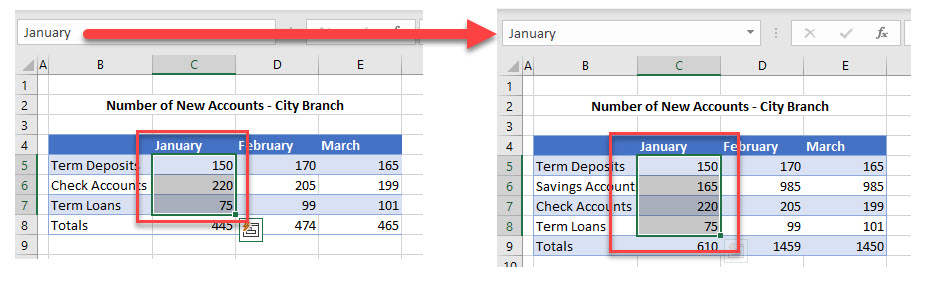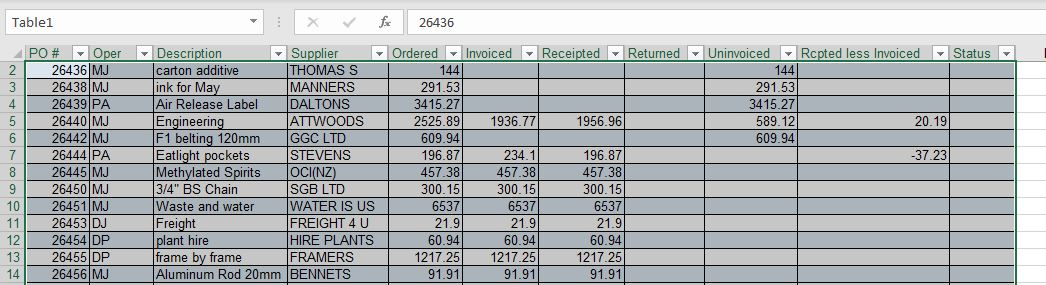# VBA Dynamic Range

This article will demonstrate how to create a Dynamic Range in Excel VBA.

Declaring a specific range of cells as a variable in Excel VBA limits us to working only with those particular cells. By declaring Dynamic Ranges in Excel, we gain far more flexibility over our code and the functionality that it can perform.

## Referencing Ranges and Cells

When we reference the Range or Cell object in Excel, we normally refer to them by hardcoding in the row and columns that we require.

### Range Property

Using the Range Property, in the example lines of code below, we can perform actions on this range such as changing the color of the cells, or making the cells bold.

``````Range("A1:A5").Font.Color = vbRed
Range("A1:A5").Font.Bold = True
``````

### Cells Property

Similarly, we can use the Cells Property to refer to a range of cells by directly referencing the row and column in the cells property. The row has to always be a number but the column can be a number or can a letter enclosed in quotation marks.

For example, the cell address A1 can be referenced as:

``Cells(1,1)``

Or

``Cells(1, "A")``

To use the Cells Property to reference a range of cells, we need to indicate the start of the range and the end of the range.

For example to reference range A1: A6 we could use this syntax below:

``Range(Cells(1,1), Cells(1,6)``

We can then use the Cells property to perform actions on the range as per the example lines of code below:

``````Range(Cells(2, 2), Cells(6, 2)).Font.Color = vbRed
Range(Cells(2, 2), Cells(6, 2)).Font.Bold = True``````

## Dynamic Ranges with Variables

As the size of our data changes in Excel (i.e. we use more rows and columns that the ranges that we have coded), it would be useful if the ranges that we refer to in our code were also to change.  Using the Range object above we can create variables to store the maximum row and column numbers of the area of the Excel worksheet that we are using, and use these variables to dynamically adjust the Range object while the code is running.

For example

``````Dim lRow as integer
Dim lCol as integer
lRow  = Range("A1048576").End(xlUp).Row
lCol  = Range("XFD1").End(xlToLeft).Column
``````

### Last Row in Column

As there are 1048576 rows in a worksheet,  the variable lRow will go to the bottom of the sheet and then use the special combination of the End key plus the Up Arrow key to go to the last row used in the worksheet – this will give us the number of the row that we need in our range.

### Last Column in Row

Similarly, the lCol will move to Column XFD which is the last column in a worksheet, and then use the special key combination of the End key plus the Left Arrow key to go to the last column used in the worksheet – this will give us the number of the column that we need in our range.

Therefore, to get the entire range that is used in the worksheet, we can run the following code:

``````Sub GetRange()
Dim lRow As Integer
Dim lCol As Integer
Dim rng As Range
lRow = Range("A1048576").End(xlUp).Row
'use the lRow to help find the last column in the range
lCol = Range("XFD" & lRow).End(xlToLeft).Column
Set rng = Range(Cells(1, 1), Cells(lRow, lCol))
'msgbox to show us the range
MsgBox "Range is " & rng.Address
End Sub``````

Stop searching for VBA code online. Learn more about AutoMacro - A VBA Code Builder that allows beginners to code procedures from scratch with minimal coding knowledge and with many time-saving features for all users!## SpecialCells – LastCell

We can also use SpecialCells method of the Range Object to get the last row and column used in a Worksheet.

``````Sub UseSpecialCells()
Dim lRow As Integer
Dim lCol As Integer
Dim rng As Range
Dim rngBegin As Range
Set rngBegin = Range("A1")
lRow = rngBegin.SpecialCells(xlCellTypeLastCell).Row
lCol = rngBegin.SpecialCells(xlCellTypeLastCell).Column
Set rng = Range(Cells(1, 1), Cells(lRow, lCol))
'msgbox to show us the range
MsgBox "Range is " & rng.Address
End Sub``````

## UsedRange

The Used Range Method includes all the cells that have values in them in the current worksheet.

``````Sub UsedRangeExample()
Dim rng As Range
Set rng = ActiveSheet.UsedRange
'msgbox to show us the range
MsgBox "Range is " & rng.Address
End Sub``````

## CurrentRegion

The current region differs from the UsedRange in that it looks at the cells surrounding a cell that we have declared as a starting range (ie the variable rngBegin in the example below), and then looks at all the cells that are ‘attached’ or associated to that declared cell.   Should a blank cell in a row or column occur, then the CurrentRegion will stop looking for any further cells.

``````Sub CurrentRegion()
Dim rng As Range
Dim rngBegin As Range
Set rngBegin = Range("A1")
Set rng = rngBegin.CurrentRegion
'msgbox to show us the range
MsgBox "Range is " & rng.Address
End Sub``````

If we use this method, we need to make sure that all the cells in the range that you require are connected with no blank rows or columns amongst them.

VBA Programming | Code Generator does work for you!

## Named Range

We can also reference Named Ranges in our code. Named Ranges can be dynamic in so far as when data is updated or inserted, the Range Name can change to include the new data.

This example will change the font to bold for the range name “January”

``````Sub RangeNameExample()
Dim rng as Range
Set rng = Range("January")
rng.Font.Bold = = True
End Sub``````

As you will see in the picture below, if a row is added into the range name, then the range name automatically updates to include that row.Should we then run the example code again, the range affected by the code would be C5:C9 whereas in the first instance it would have been C5:C8.

## Tables

We can reference tables (click for more information about creating and manipulating tables in VBA) in our code. As a table data in Excel is updated or changed, the code that refers to the table will then refer to the updated table data.  This is particularly useful when referring to Pivot tables that are connected to an external data source.Using this table in our code, we can refer to the columns of the table by the headings in each column, and perform actions on the column according to their name.  As the rows in the table increase or decrease according to the data, the table range will adjust accordingly and our code will still work for the entire column in the table.

For example:

``````Sub DeleteTableColumn()
ActiveWorkbook.Worksheets("Sheet1").ListObjects("Table1").ListColumns("Supplier").Delete
End Sub``````